# DAV Class 3 Maths Chapter 5 Worksheet 7 Solutions

The DAV Class 3 Maths Book Solutions and DAV Class 3 Maths Chapter 5 Worksheet 7 Solutions of Division offer comprehensive answers to textbook questions.

## DAV Class 3 Maths Ch 5 WS 7 Solutions

Question 1.
Divide

(a)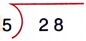Solution:Quotient = 5
Remainder = 3

(b)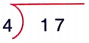Solution: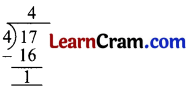Quotient = 4
Remainder = 1

(c)Solution:Quotient = 6
Remainder = 1(d)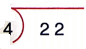Solution:Quotient = 5
Remainder = 2

(e)Solution: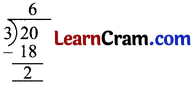Quotient = 6
Remainder = 2

(f)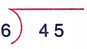Solution: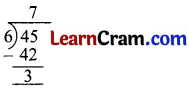Quotient = 7
Remainder = 3(g)Solution: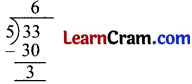Quotient = 6
Remainder = 3

(h)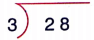Solution: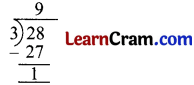Quotient = 9
Remainder = 1

(i)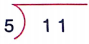Solution: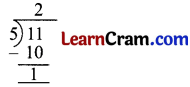Quotient = 2
Remainder = 1### DAV Class 3 Maths Chapter 5 Worksheet 7 Notes

32 ÷ 6

1. Recite the table of 6.
2. Recite till you get the number just less than 32 as you will not get 32 in table.
3. Now subtract the product from dividend. You get 2.